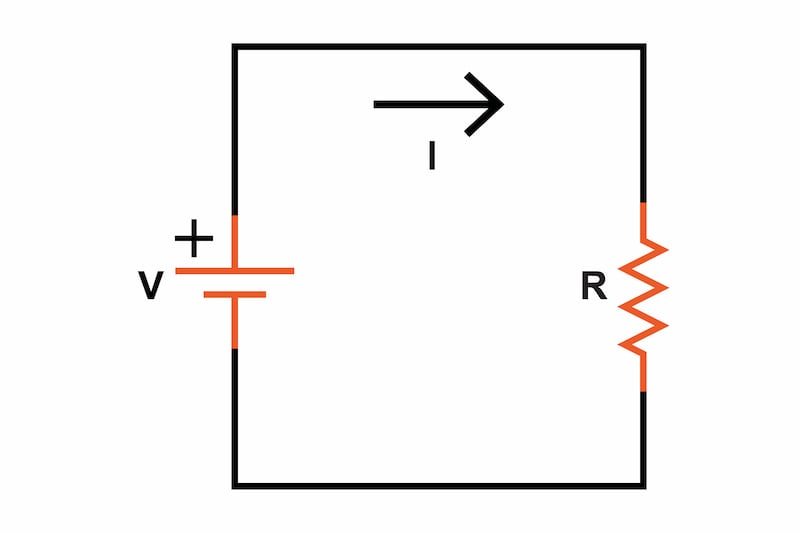# Electrical Energy Calculator

## This calculator will determine the instantaneous energy, sustained energy, and power consumed by an electrical system given two of the three electrical parameters (voltage, current, or resistance) and the time.

Inputs Value Units
Outputs Value Units

Knowing the power and energy consumed by a device can be important when determining the system temperature increase or other thermal considerations when designing electronic circuits. This calculator may also be useful when finding the energy consumption of a circuit to determine the battery life or choosing the correct size battery for a device.### Electrical Energy Equation:

We can use any of the following equations to find the power dissipated by a resistive circuit element.

$$P = VI = I^2R = \frac{V^2}{R}$$

Where:

• P = Power dissipated in a resistive element in watts
• V = The voltage drop across the resistive element in volts
• I = The current through the resistive element in amps

### Joule's Law Equation for Energy Consumption

From here, we can find the total energy consumed by the circuit by using the following equation (also known as Joule’s Law):

$$E = Pt$$

Where:

• E = Energy dissipated in a resistive element in joules
• P = Power dissipated in a resistive element in watts
• t = The duration of time the energy was dissipated in seconds

By definition 1 watt = 1 joule per second (1 W = 1 J/s). Similarly, you can flip that equation around and it becomes 1 joule = 1 watt for 1 second (1 J = 1 w-s)

### Converting Watt-hours to Joules

It's also important to note that energy is also commonly expressed in terms of watt-hours which, as the name suggests, is the energy equivalent of one watt of power consumed continuously for one hour. One watt-hour is equal to 3600 Joules (3.6 kJ). Below is a quick conversion equation.

$$Wh = \frac{E}{3600}$$

Where:

• Wh = Energy consumed for a period of time in watt-hours
• E = Energy dissipated in a resistive element in joules